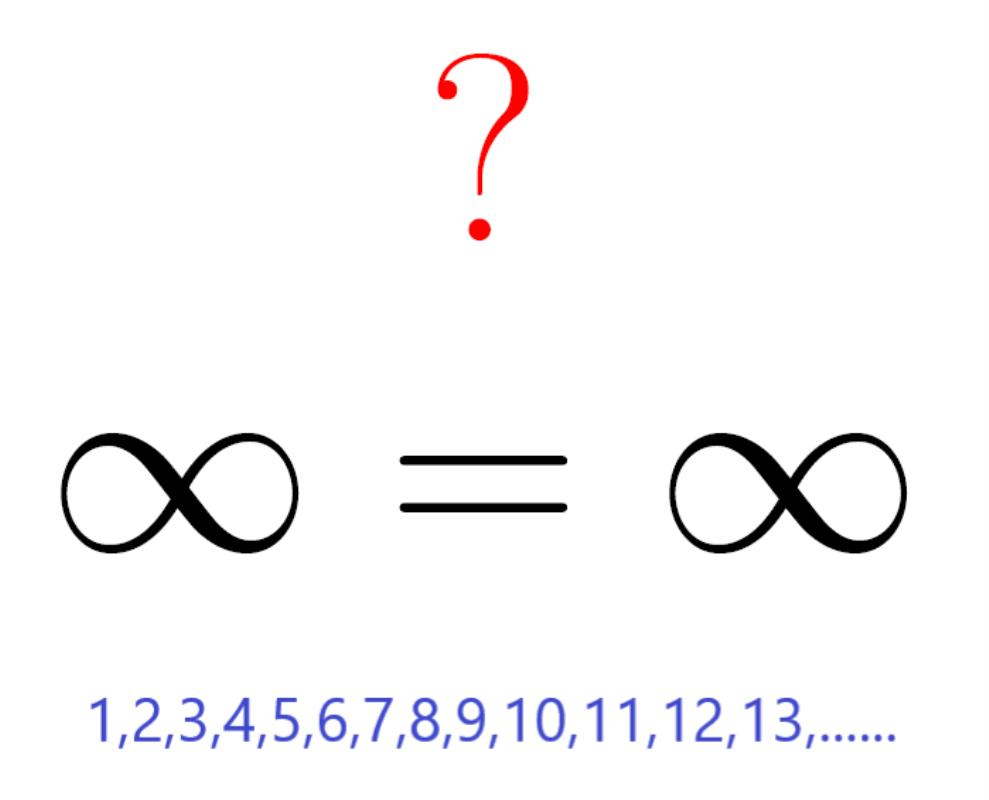# Is infinity equal to infinity?It doesn’t take long for a child to grasp the notion of infinity. If you ask a 5 year old what the largest number in the world is, the child knowing only a handful of numbers might say a small number like 15.

As the child grows older, he/she learns more numbers into the hundreds and quickly realizes that for every large number he/she can come up with, say 120, there is a larger one, say 121, so we cannot find a largest number.

This is how one usually understands the notion of infinity.

What is interesting is that there are different “types” of infinity. To explain this, let’s discuss different sets of numbers

• The natural numbers are the numbers 0, 1, 2, 3, 4,…
• The integers are the same as the natural numbers but with negative values too. For example, -9, -7, 6, 9
• The rational numbers are all the fractions composed from integers. For example, ½, -⅔, 5=5/1
• The real numbers are even a bigger set than the rational numbers consisting of numbers like √ ̅2, ⅞, π

Certainly each of the above sets are infinite. However, the first three sets above have the same “type” of infinity whereas the fourth is different. Mathematicians say that a set is countable if it has the same type of infinity as the natural numbers. The word countable is probably not the best chosen word here; listable is a better word. What it means for a set to be listable (or countable) is that it is possible to write down all the elements of the set in a list.

The natural numbers are listable because we can write them as 0,1,2,3,4,…. i.e. in a list. We can write the integers in the following way 0,-1,1,-2,2,-3,3,…. which shows that they are listable. The rational numbers can also be put into a list, but I will not explain here how this is done.

The notion of countable/listable was proposed by the German mathematician Georg Cantor in the late 19th century. He was the first mathematician to take a systematic look at infinity. After understanding that the first three sets above were all listable, Cantor asked whether the real numbers were listable too.

If you were to attempt to do this, you would realize quickly that to find a strategy to list all the real numbers is difficult. You might try as a first attempt to go like this 1, 1.1, 1.2, 1.3, 1.4, 1.5, 1.6, 1.7, 1.8, 1.9, 2, 2.1, 2.2, 2.3,…. but then you realize that you missed 1.11.

You can insert it in your list somewhere, but then again you notice that you missed 1.111 so you insert it. Can you keep inserting the missing numbers to create a list?

It seems not, but you need to produce a convincing argument as to why not.

In 1874 Georg Cantor proved that the real numbers are not listable. His proof is very beautiful and actually not very difficult to understand. To keep this article nontechnical, I will not attempt to explain the proof here.

Cantor’s proof was a very big breakthrough in mathematics. He showed that not all infinities are the same; the first three sets above have the same type of infinity because they are listable but the real numbers have a bigger type of infinity because they are not listable.

If we call the type of infinity of the natural numbers A and the type of infinity of the real numbers B, then Cantor’s proof shows that A < B.

The interesting question asked by Cantor after he proved that A < B is whether there exists a set of infinity type C such that A < C < B i.e. if there is a set whose “infinity size” is larger than the size of the natural numbers but smaller than the real numbers.

This question became a central question in mathematics for many years. Cantor himself could not answer it.

The final answer to this question came in 1963 by mathematician Paul Cohen complementing earlier work of mathematician Kurt Godel in 1940.

The answer strangely enough is that we cannot prove nor disprove that there is a set with “infinity type” C such that A < C < B. Try to wrap your head around that! The statement is on the border between mathematics and philosophy.

# Author Details

Articles

Followers

## 0

Following

Assistant Professor, Dept. Of Mathematics, University of Bahrain